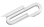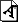Spring 2020

# April 14

Questions about momentum ?

The rest of the term:

We have three full weeks counting this one, then a last Tues May 5 class. My plan is to spend two weeks on rotations - expanding the material in the texts chap 12 with some outside readings - and then one week on gravity and planetary motion, chap 13.

If you want a grade for the class, then you should start thinking about doing a second review paper, with a worked exemplar problem for the second half of the term, chapters 9 (energy), 10 (more energy), 11 (momentum), 12 (rotation), and 13 (gravity). That will be due Fri May 9.

If you're doing the class pass/fail, just continue on with the weekly assignments.

TLDR - rest of semester schedule

this week of Apr 14 : chapter 12, rotations
next week of Apr 21 : more rotations, 3D & static equlibrium
following    Apr 28 : motion of the planets
last class   May 5  : review and/or something fun


# rotations

I've posted a starting point with some notes with some of the essential definitions and formulas, and we'll start there and with the chap 12 slides

## sources

our text :

• chap 12, Rotation of a Rigid Body

openstax University Physics Volume 1

Kleppner & Kolenkow :

• chap 6 : Angular Momentum and Fixed Axis Rotation (2D)
• chap 7 : Rigid Body Motion and the Conversation of Angular Momentum (3D)
• 8.012 MIT course : problem sets 6, 7, 8 (or parts of those)

Feynman lectures : chap 18, 19, 20 :

wikipedia :

## nitpicky notes (for after you've looked at definitions and readings)

• kinetic energy has linear and rotational parts; they add. It's all one total energy.
• linear momentum and angular momentum are two different things, each may be conserved depending on the conditions.
• the definition of torque and angular momentum depend on where the origin is.
• Often we use the center of mass ... but sometimes another origin gives other insights.
• a particle moving in a straight line has angular momentum, if the origin is not on that line
• angular velocity is in 3D a "pseudo vector" $$\overrightarrow{\omega}$$ along the rotation axis (right hand rule).
• the angular momentum $$\overrightarrow{\omega}$$ in 3D is not always parallel to $$\overrightarrow{\omega}$$ .
• the moment of inertia I is in the 3D general case a tensor - a 3x3 matrix of numbers. See for example wikipedia: inertia tenso
https://cs.marlboro.college /cours /spring2020 /mechanics /notes /apr14
last modified Mon September 26 2022 2:27 am

## attachmentslast modified sizecross_product.pdf Mon Sep 26 2022 02:27 am 486Kjims_rotation_notes.pdf Mon Sep 26 2022 02:27 am 449Kproblem_11_51.pdf Mon Sep 26 2022 02:27 am 332Kright_hand_rule.pdf Mon Sep 26 2022 02:27 am 174Krotation_formula_summary.pdf Mon Sep 26 2022 02:27 am 449K